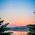## Thursday, February 26, 2015

### 53 86 | The Death of Earl Lloyd, NBA's First Black Player

• Earl = 5+1+9+3 = 18
• Lloyd = 3+3+6+7+4 = 23
• Earl Lloyd = 41 = 5
• Earl = 5+1+18+12 = 36
• Lloyd = 12+12+15+25+4 = 68
• Earl Lloyd = 104 = 5
Earl Lloyd is a good name for the 'old 5 ball'.  He was the first black player in the professional basketball league.  Notice his initials are EL, or '53', having much to do with black athletes dying in their 80s, from Ernie Banks to Earl Lloyd.
• EL = 53
Dying at the age of 86 is also curious...
• Triangle = 86
• Pyramid = 86

#### 2 comments:

1.Earl 'The Pearl' = 121

1.Moon Fixer = 119